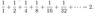# Convergence for a series

• soopo

## Homework Statement

You can see the problem in the attachment.

## The Attempt at a Solution

I am not sure why the series converges.
It does not make sense, since I apparently could form halves from the terms recursively, similarly as for the proof of harmonic series' divergence.

How can you prove that the series converges?

#### Attachments

•Picture 3.png
1.5 KB · Views: 386
It's a geometric series with common ratio 1/2

That's why it converges

soopo said:
It does not make sense, since I apparently could form halves from the terms recursively, similarly as for the proof of harmonic series' divergence.

Can you explicitly show this grouping into halves?

Hi soopo!soopo said:
… I apparently could form halves from the terms recursively, similarly as for the proof of harmonic series' divergence.

I'm not sure what you mean by that, but if for example you call the sum A, then A/2 = A - 1 (the first term), so A/2 = 1How can you prove that the series converges?

Easiest way is to notice that the first n terms are 1 + … + xn-1, which you should recognise.slider142 said:
Can you explicitly show this grouping into halves?

You are right - it is hard to show that.

For example,

1/4 + 1/8 + 1/16 + 1/32 = 13/32 < 16/32 = 1/2

By continuing similarly, I guess we can form another value which is close to an half, since the series goes to infinity.

However, I cannot find the second term which is close to a half, since it would require apparently much more terms than the second term.
This way, the third term also seems to be extremely difficult to be obtained.

JG89 said:
It's a geometric series with common ratio 1/2

That's why it converges

True!

I know that the sum of geometric series is
S = a(1 - r^n) / (1 - r),
where r is not 1.

It can be easily obtained by considering to consider the series A and rA where

A = 1 + r + r^2 + ...

Then, substacting rA from A, we get

(1 - r)A =1 - $$r^{n + 1}$$,
since most of the terms in the middle cancel out.

If
-1 < r < 1, then r^n -> 0 as n -> infinity.
This gives us the sum of the series to be
S = a/(1 - r).

So my mistake was that I did not consider the series as a geometric series. I wanted to show that you can use a similar proof of harmonic series' divergence for the geometric series, since they both have same terms.

However, as we have seen, it is impossible to show that the geometric series diverges when |r| < 1. Thus, the series converges to 2.

soopo said:
You are right - it is hard to show that.

For example,

1/4 + 1/8 + 1/16 + 1/32 = 13/32 < 16/32 = 1/2

By continuing similarly, I guess we can form another value which is close to an half, since the series goes to infinity.

However, I cannot find the second term which is close to a half, since it would require apparently much more terms than the second term.
This way, the third term also seems to be extremely difficult to be obtained.

In fact, it cannot be obtained. ;) In order to group by halves, you would have to show a sum that is at least equal to a half, not less than a half. You have instead given a good argument for the sum to converge by showing an upper bound (with a little more rigor). As the others have posted, this is an example of a geometric series; the terms shrink "too fast" to reach the 1/2 and converge easily.

This is how a (physics) Prof. in a class I attended a long time ago proved it. He said: Look, I'm standing here now and then he jumped and said: Now that's a big step of two meters. But I can cover the same distance by doing a step that covers half the distance, i.e. one meter and then another step of one meter.

But wait, I could just as well cut that second step of one meter in half and then two two steps of half a meter instead of one step of one meter. But that last step of half a meter can also be cut in half etc. etc. If we always do a step that is half of the distance we need to go, we'll reach the destination in the limit. So, we have:

2 = 1 + 1 = 1 + 1/2 + 1/2 = 1 + 1/2 + 1/4 + 1/4 = 1 + 1/2 + 1/4 + 1/8 + 1/8 = ...

Count Iblis said:
This is how a (physics) Prof. in a class I attended a long time ago proved it. He said: Look, I'm standing here now and then he jumped and said: Now that's a big step of two meters. But I can cover the same distance by doing a step that covers half the distance, i.e. one meter and then another step of one meter.

But wait, I could just as well cut that second step of one meter in half and then two two steps of half a meter instead of one step of one meter. But that last step of half a meter can also be cut in half etc. etc. If we always do a step that is half of the distance we need to go, we'll reach the destination in the limit. So, we have:

2 = 1 + 1 = 1 + 1/2 + 1/2 = 1 + 1/2 + 1/4 + 1/4 = 1 + 1/2 + 1/4 + 1/8 + 1/8 = ...

Excellent analogy to the reverse direction!